# Ranking Functions in SQL Server

Total: 10 Average: 4.6

Suppose you are designing an SQL Server database application for a company’s CEO and you have to display the fifth most highly paid employee in the company.

What would you do? One solution is to write a query like this:

```SELECT EmployeeName
FROM Employees
ORDER BY Salary DESC
OFFSET 4 ROWS
FETCH FIRST 1 ROWS ONLY;```

The query above looks cumbersome, particularly if you have to rank all of the employees. In that case, one solution is to list the employees by descending order of salary and then take the index of the employee as the rank. However, things get complicated if the multiple employees have the same salary. How would you rank them?

Fortunately, SQL Server comes with built-in ranking functions that can be used to rank records in a variety of ways. In this article, we will introduce SQL server ranking functions in detail illustrating it with the examples.

There are four different types of ranking function in SQL Server:

• Rank()
• Dense_Rank()
• Row Number()
• Ntile()

It is important to mention that all the ranking functions in SQL server require the ORDER BY clause.

Before we look at each of the ranking functions in detail, first, let us create dummy data that we will be using in this article to explain the ranking function. Execute the following script:

```CREATE DATABASE Showroom

Use Showroom
CREATE TABLE Car
(
CarId int identity(1,1) primary key,
Name varchar(100),
Make varchar(100),
Model int ,
Price int ,
Type varchar(20)
)

insert into Car( Name, Make, Model , Price, Type)
VALUES ('Corrolla','Toyota',2015, 20000,'Sedan'),
('Civic','Honda',2018, 25000,'Sedan'),
('Passo','Toyota',2012, 18000,'Hatchback'),
('Land Cruiser','Toyota',2017, 40000,'SUV'),
('Corrolla','Toyota',2011, 17000,'Sedan'),
('Vitz','Toyota',2014, 15000,'Hatchback'),
('Accord','Honda',2018, 28000,'Sedan'),
('7500','BMW',2015, 50000,'Sedan'),
('C200','Mercedez',2010, 26000,'Sedan'),
('Corrolla','Toyota',2014, 19000,'Sedan'),
('Civic','Honda',2015, 20000,'Sedan')```

In the script above, we create Showroom database with one table Car. The Car table has five attributes: CarId, Name, Make, Model, Price and Type.

Next, we added 12 dummy records in the Car table.

Now, you see each of the ranking functions.

### 1. Rank Function

The rank function in SQL server assigns rank to each record ordered by the ORDER BY clause. For instance, if you want to see the fifth most expensive car in the Car table, you can use the rank function as follows:

```Use Showroom
SELECT Name,Make,Model, Price, Type,
RANK() OVER(ORDER BY Price DESC) as PriceRank
FROM Car```

In the script above, select the Name, Make, Model, Price, Type and the rank of each car ordered by Price as the “PriceRank” column. The syntax for Rank function is simple. You have to write the function RANK followed by the OVER operator. Inside the OVER operator, you need to pass the ORDER BY clause that sorts the data. The output of the script above looks like this: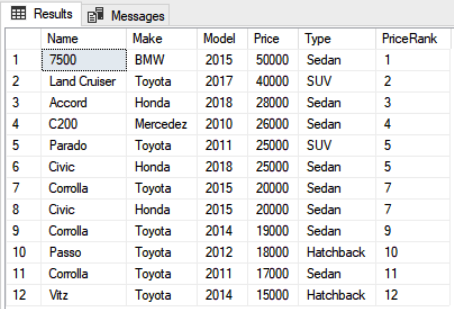You can see the rank for each car. It is important to mention that if there is a tie between the ranks of two records, the next ranking position is skipped. For instance, there is a tie between record 5 and 6 in the output. Both Parado and Civic have equal prices, and hence have been ranked 5. However, the next rank, in particular, rank 6 is skipped and the next two cars in the list have been ranked 7 since they also have same price. After the 7th rank, the rank 8 is skipped again and the next assigned rank is 9.

You can divide the data into partitions and then apply ranking to individual partitions. In the following script, there is the partition of the records by type. We rank the cars inside each partition.

```SELECT Name,Make,Model, Price, Type,
RANK() OVER(PARTITION BY Type ORDER BY Price DESC) as PriceRank
FROM Car```

The output of the script above looks like this: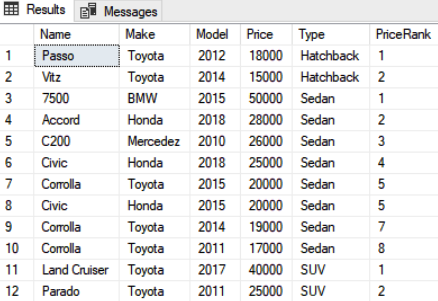It is evident from the output that the records have been partitioned according to car types and the rank has been assigned locally inside the partition. For instance, the first two records belong to partition “Hatchback” and have been ranked 1 and 2. For the next partition, i.e. “Sedan”, the rank is reset to 1.

### 2. Dense_Rank Function

The dense_rank function is similar to the rank function. However, in case of dense_rank, if there is a tie between two records in terms of the rank, the next rank is not skipped. Let’s see demonstrate it with the example. Execute the following script:

```Use Showroom
SELECT Name,Make,Model, Price, Type,
DENSE_RANK() OVER(ORDER BY Price DESC) as DensePriceRank
FROM Car```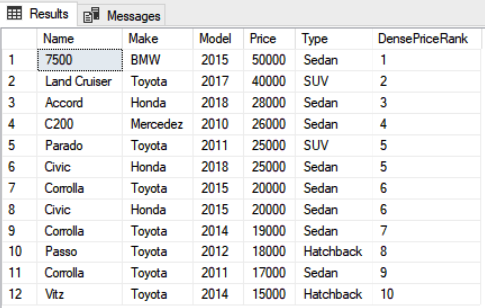Again you can see that the 5th and 6th record has the same value for Price and both have been assigned rank 5. However, unlike rank function that skipped the next rank, the dense_rank function doesn’t skip the next rank, and the rank 6 has been assigned to the next record.

Like rank function, the dense_rank function can also be applied to the partition by clause. Look at the following script:

```SELECT Name,Make,Model, Price, Type,
DENSE_RANK() OVER(PARTITION BY Type ORDER BY Price DESC) as DensePriceRank
FROM Car```

The output of the script above looks like this: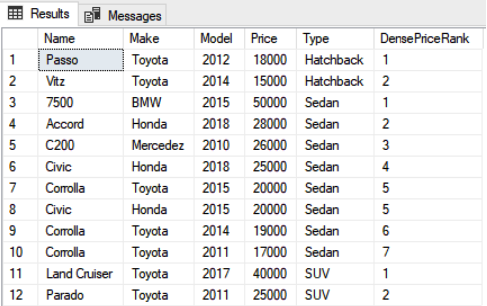### 3. Row_Number Function

The row_number function also ranks the records according to the conditions specified by the ORDER BY clause. However, unlike the rank and dense_rank functions, the row_number function doesn’t assign same rank where there are duplicate values for the column specified by the ORDER BY clause. Look at the following script:

```SELECT Name,Make,Model, Price, Type,
DENSE_RANK() OVER(PARTITION BY Type ORDER BY Price DESC) as DensePriceRank
FROM Car```

The output of the script above looks like this: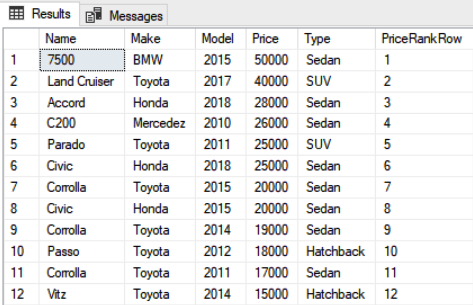From the script above, you can see that both 5th and 6th records have the same value for Price column, but the rank assigned to them is different.

Similarly, the row_number function can be applied to the partitioned data. Look at the following script for example.

```SELECT Name,Make,Model, Price, Type,
ROW_NUMBER() OVER(PARTITION BY Type ORDER BY Price DESC) AS PriceRankRow
FROM Car```

The output of the script above looks like this: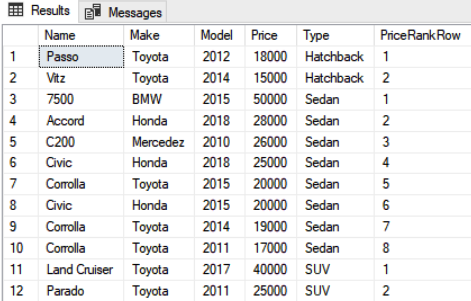### 4. NTILE Function

NTILE function groups the ranking. Suppose you have 12 records in a table, and you want to rank them into groups of 4. The first three records will have rank 1, the next three records will have rank 2 and so on.

Let’s take a look at an example of the NTILE function.

```Use Showroom
SELECT Name,Make,Model, Price, Type,
NTILE(4) OVER(ORDER BY Price DESC) as NtilePrice
FROM Car```

In the above script, we passed 4 as a parameter to the NTILE function. Since we have 12 records, you will see a total of 4 different ranks where 1 rank will be assigned to three records. The output looks like this: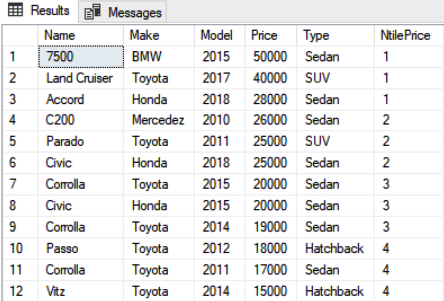You can see that the first three most expensive cars have been ranked 1, the next three have been ranked 2 and so on.

The NTILE function can also be applied to the partitioned data. Look at the following script:

```SELECT Name,Make,Model, Price, Type,
NTILE(4) OVER(PARTITION BY Type ORDER BY Price DESC) as NtilePrice
FROM Car```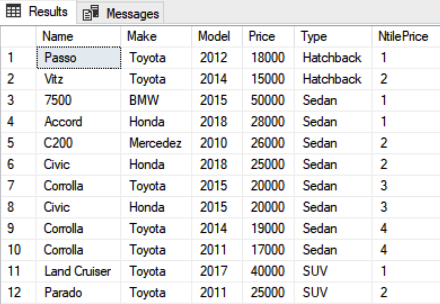### Conclusion

Ranking functions in SQL Server are used to rank data in different ways. In this read, we introduced different types of ranking functions with the examples. The rank and dense_rank functions give same rank to the data with same values in the ORDER BY clause whereas the row_number function ranks the record in incremental way even if there is a tie.
In case of no duplicate records in the column specified by the ORDER BY clause, rank, dense_rank and row_number functions behave in a similar manner.#### Ben Richardson

Ben Richardson runs Acuity Training. Acuity is an IT training business offering classroom courses in London and Guildford. It is a leading provider of SQL training the UK and offers a full range of SQL training from introductory training to advanced administration courses.